# 12. Mechanical design / Machine design¶

## Instruction¶

### 1.1 Mechanical Design (part 1 of 2)¶

Group assignment: Design a machine that includes mechanism + actuation + automation Build the mechanical parts and operate it manually. Document the group project -> FabLab Armenia Dilijan and Fablab Kannai Group Page

### 1.2 Machine Design (part 2 of 2)¶

Group assignment:Actuate and automate your machine. Document the group project -> FabLab Armenia Dilijan and Fablab Kannai Group Page

## Briefing¶

We decided to collaborate with Dilijian, Armenia for this assignment.

First we created a group in Mattermost to keep in touch and to upload different content for us.

We started dividing the time with a schedule, so we would have a tidy process.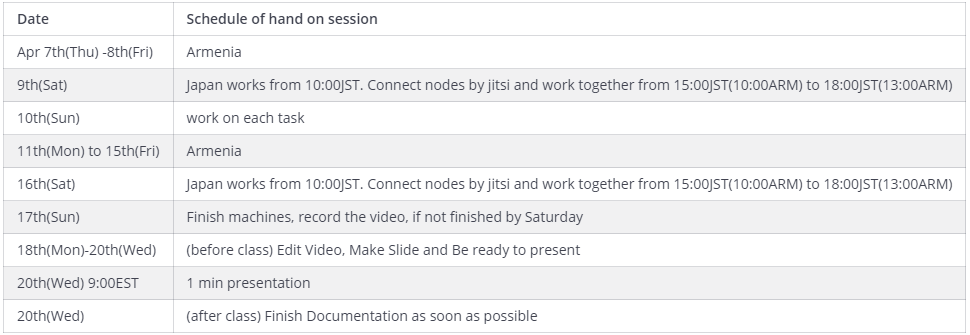And as well, we had regular meetingsx

## Final Product¶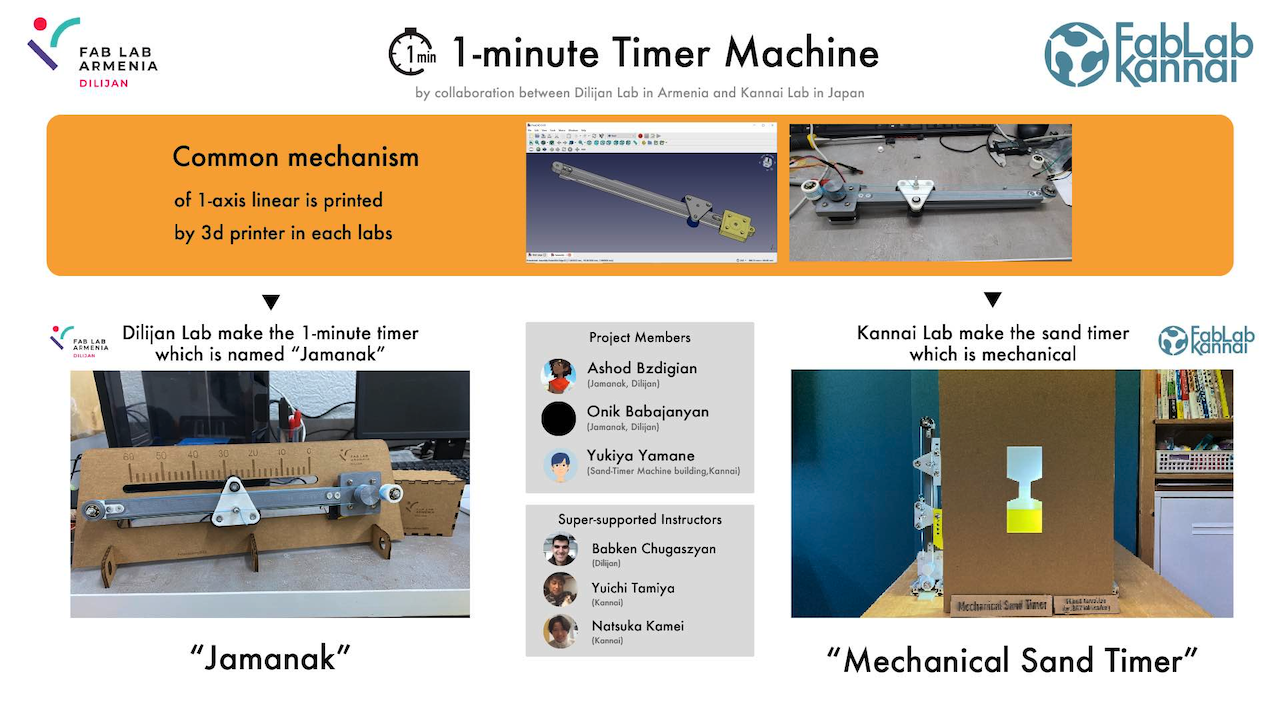Details are written in **Dilijian-Kannai Group Site.**

## Design Process¶

It was decided that the 1 liniar axis moving parts to be output by 3D printing would be common parts with the lab. We decided to use that common component to build a timer that would measure one minute in each of the two labs.

### Building Process : Common parts with Dilijian Lab¶

• Our common parts of 1-axis linear between in Dilijan lab and in Kannai lab
• which are 3d printable parts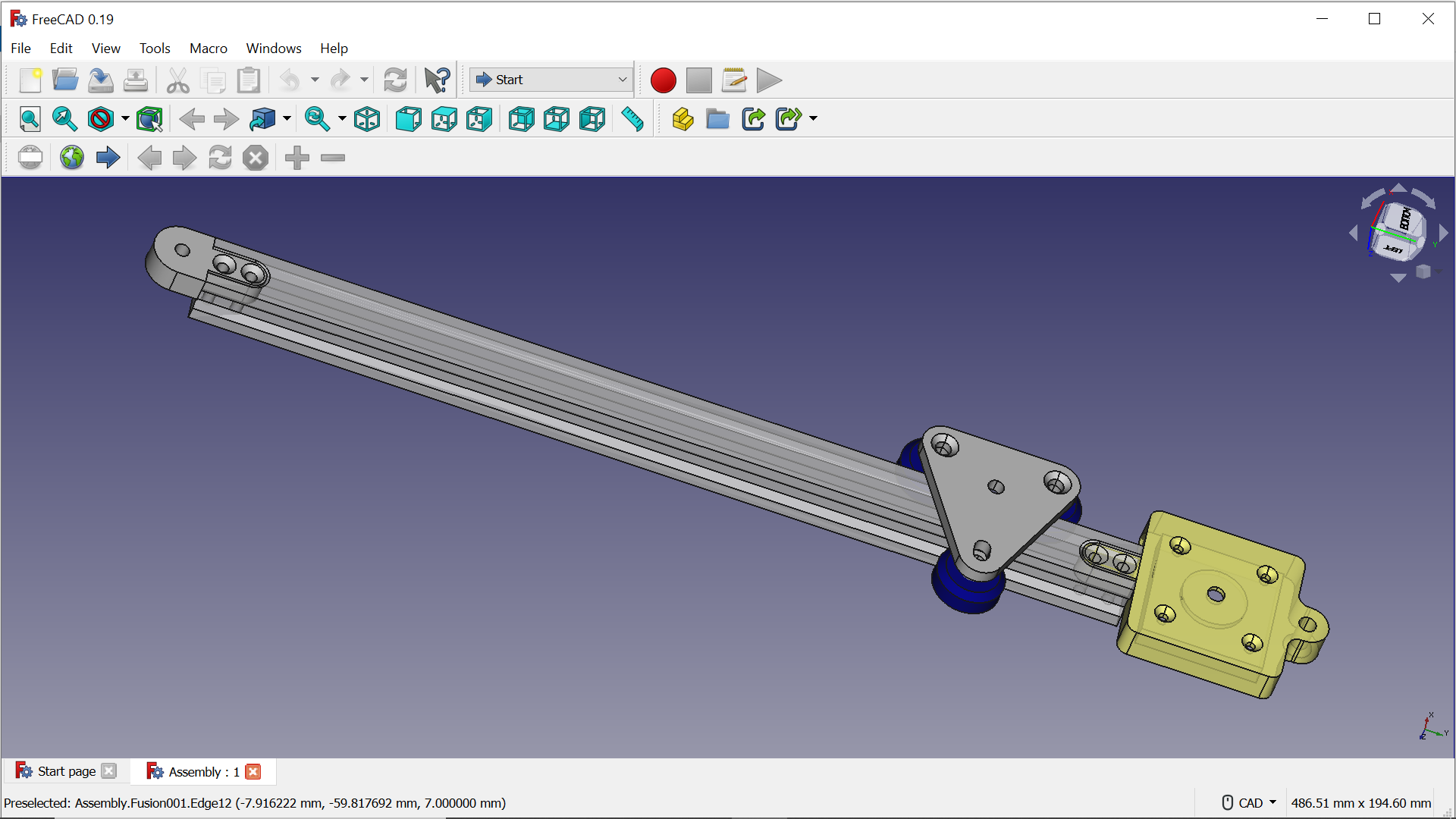• We usedCommon axis parts by 3d printer

Extrusion Rail (Meshed)_250mm.stl

bearingpullerpassthrough.stl

Extrusion Rail (Meshed).stl

capstan_pulley (1).stl

Stepper mount (Meshed).stl

Carrier with ribs (Meshed).stl

capstan_motor (1).stl

Idler mount (Meshed).stl

Spacer002 (Meshed).stl

Due to the size limitation of the 3D printer

Extrusion rails only 280mm → 250mm

Transform 3d data to g-code by Cura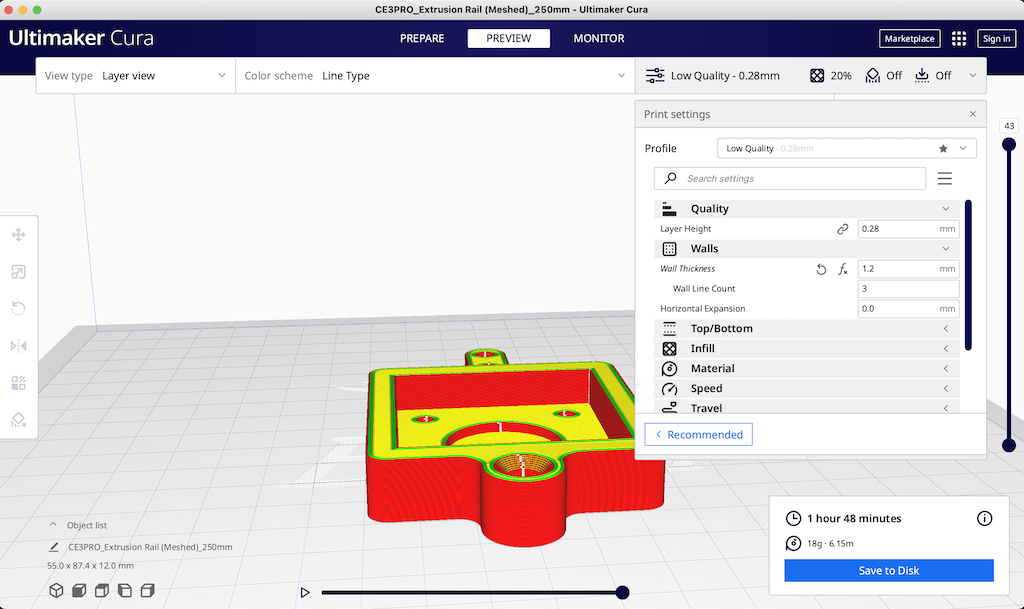Low quality

Walls Wallthickness 1.2mm

Infill 20% cubic

• 3D printed to make parts by Ender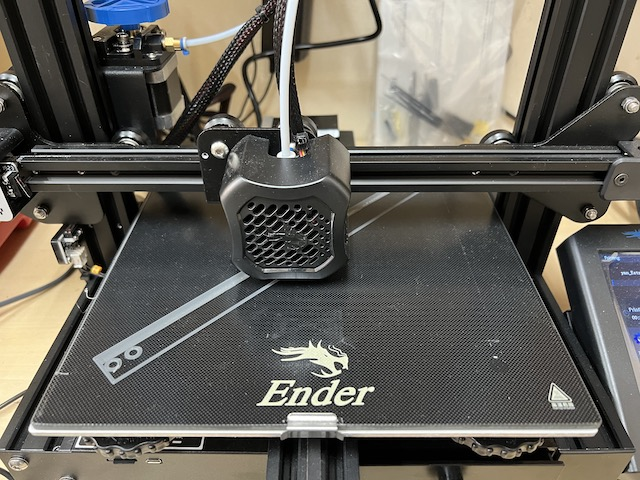• stepper motor

We decided to use this stepper motor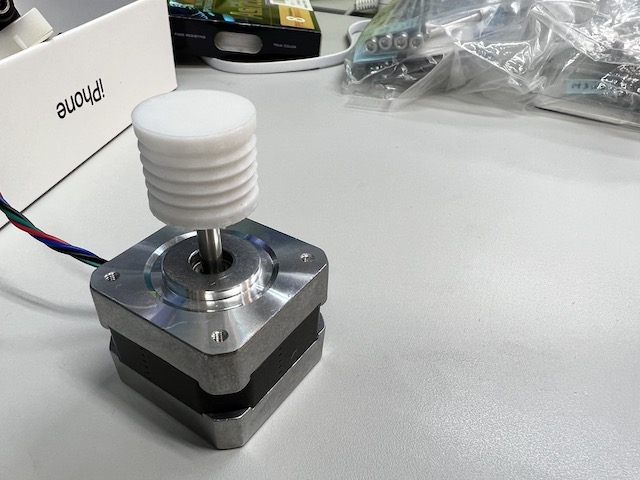• Assembling

The kite string was wired to create the mechanism carefully.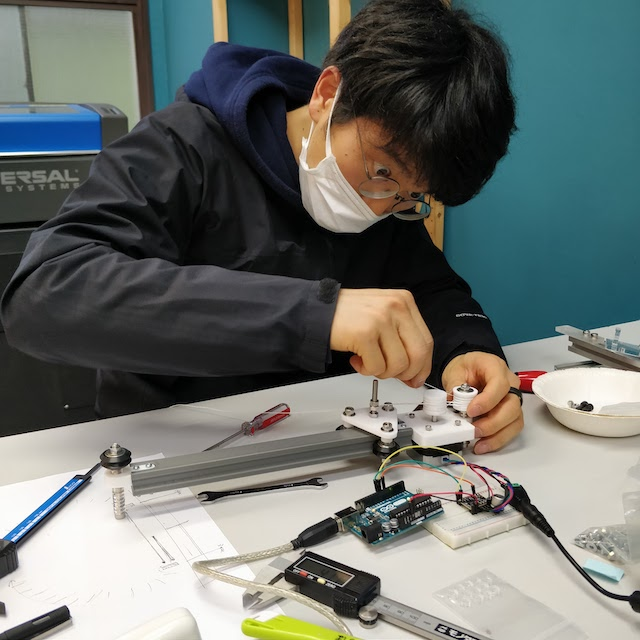• finish to make the common parts with Dilijan Lab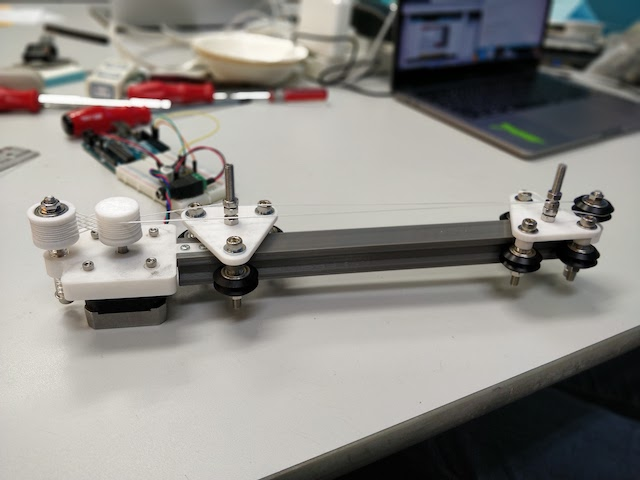• Moving Test

## Programming¶

We decided to use Arduino Uno to control the move of the stepper motor. To use it, I connected Arduino Uno to Stepper Motor Driver TMC2208 refering to this Kannai Lab’s instruction Page.

Arduino uno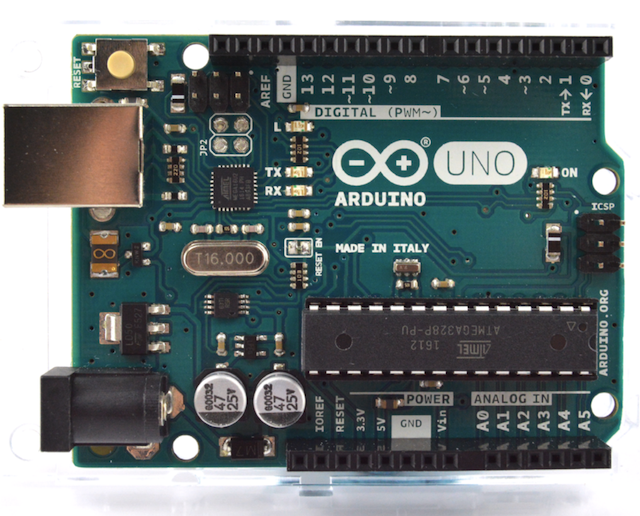Stepper motor Driver TMC 2208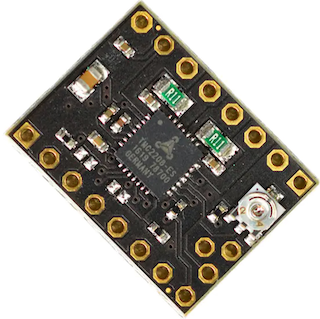Connection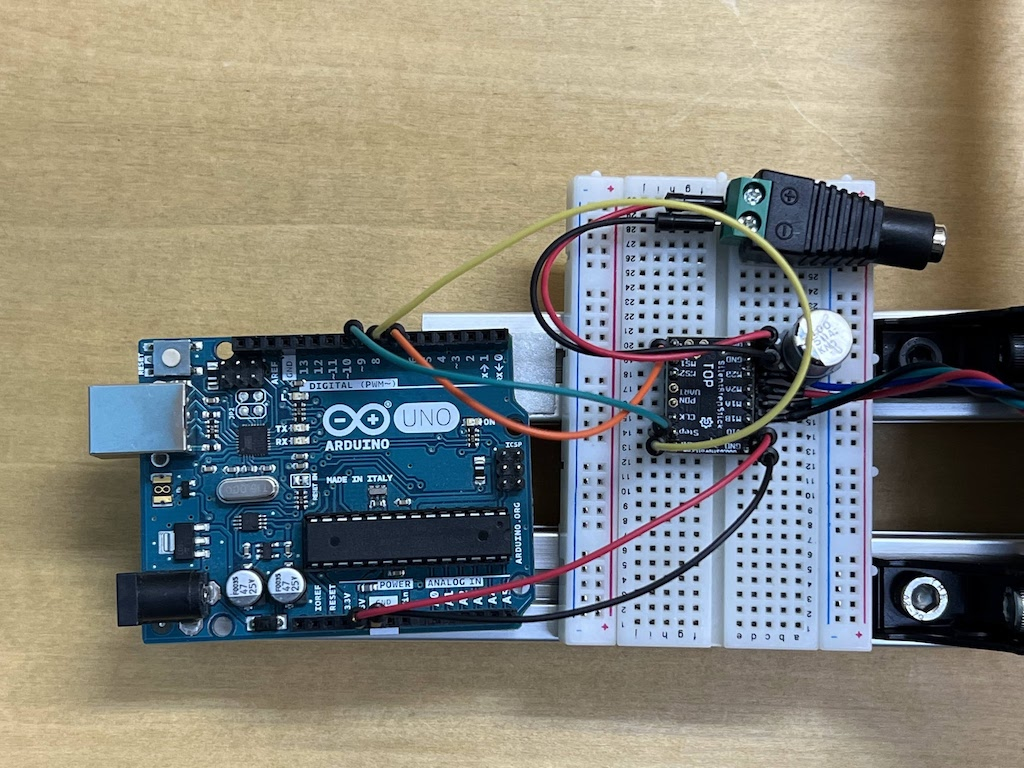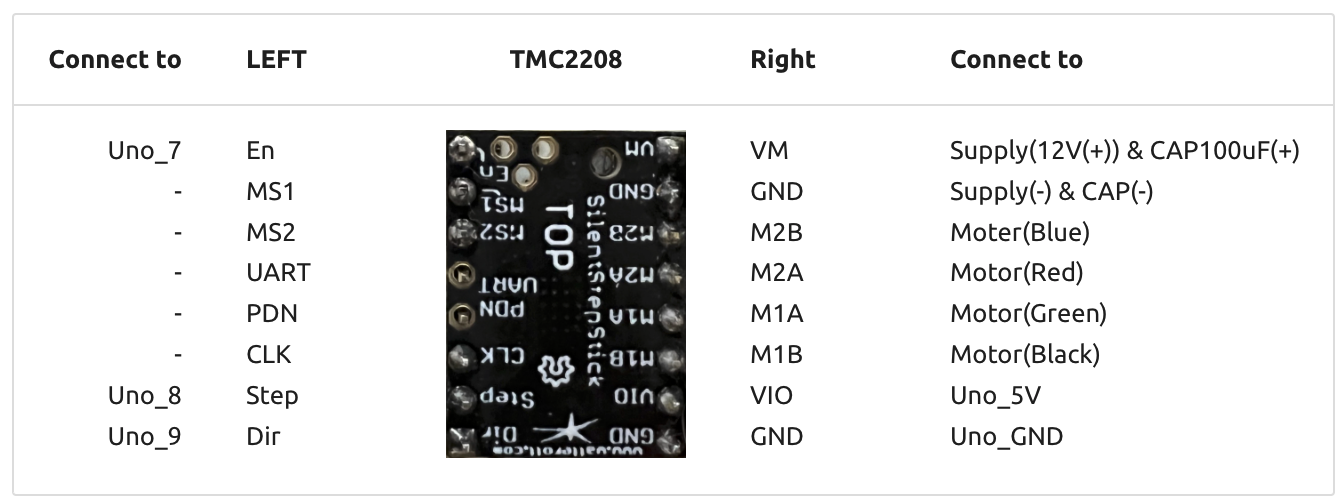### Test 1 : Move to 1 direction¶

``````/*
SilentStepStick TMC2208/TMC2209 Example
Rsense: 0.11 Ohm
Other examples/libraries can be found here:
https://github.com/teemuatlut/TMCStepper
https://github.com/trinamic/TMC-API
https://github.com/manoukianv/TMC2208Pilot
Example source code free to use.
*/

// Note: You also have to connect GND, 5V/VIO and VM.
//       A connection diagram can be found in the schematics.
#define EN_PIN    7 //enable (CFG6)
#define DIR_PIN   8 //direction
#define STEP_PIN  9 //step

void setup()
{
//set pin modes
pinMode(EN_PIN, OUTPUT);
digitalWrite(EN_PIN, HIGH); //deactivate driver (LOW active)
pinMode(DIR_PIN, OUTPUT);
digitalWrite(DIR_PIN, LOW); //LOW to CCW
//digitalWrite(DIR_PIN, HIGH); //HIGH to CW
pinMode(STEP_PIN, OUTPUT);
digitalWrite(STEP_PIN, LOW);

digitalWrite(EN_PIN, LOW); //activate driver
}

void loop()
{
//make steps
digitalWrite(STEP_PIN, HIGH);
delay(2);
digitalWrite(STEP_PIN, LOW);
delay(2);
}
``````

It worked well.

### Test 2 : Move back and forth¶

arrange code

``````/*
SilentStepStick TMC2208/TMC2209 Example
Rsense: 0.11 Ohm
Other examples/libraries can be found here:
https://github.com/teemuatlut/TMCStepper
https://github.com/trinamic/TMC-API
https://github.com/manoukianv/TMC2208Pilot
Example source code free to use.
*/

// Note: You also have to connect GND, 5V/VIO and VM.
//       A connection diagram can be found in the schematics.
#define EN_PIN    7 //enable (CFG6)
#define DIR_PIN   8 //direction
#define STEP_PIN  9 //step

void setup()
{
//set pin modes
pinMode(EN_PIN, OUTPUT);
digitalWrite(EN_PIN, HIGH); //deactivate driver (LOW active)

pinMode(DIR_PIN, OUTPUT);
//digitalWrite(DIR_PIN, LOW); //LOW to CCW
digitalWrite(DIR_PIN, HIGH); //HIGH to CW

pinMode(STEP_PIN, OUTPUT);
digitalWrite(STEP_PIN, LOW);

digitalWrite(EN_PIN, LOW); //activate driver
}

void loop()
{

for (int i = 0; i <= 255; i++){
digitalWrite(DIR_PIN, LOW); //LOW to CCW
//make steps
digitalWrite(STEP_PIN, HIGH);
delay(2);
digitalWrite(STEP_PIN, LOW);
delay(2);
}

for (int i = 0; i <= 255; i++){
digitalWrite(DIR_PIN, HIGH); //HIGH to CW
//make steps
digitalWrite(STEP_PIN, HIGH);
delay(2);
digitalWrite(STEP_PIN, LOW);
delay(2);
}

}
``````

### Test 3 : Experiment about how to proceed¶

Since the time to turn the axis = the width of movement is controlled by changing the value of i, I set the value of i appropriately and observed how many centimeters the axis moved.

``````/*
SilentStepStick TMC2208/TMC2209 Example
Rsense: 0.11 Ohm
Other examples/libraries can be found here:
https://github.com/teemuatlut/TMCStepper
https://github.com/trinamic/TMC-API
https://github.com/manoukianv/TMC2208Pilot
Example source code free to use.
*/

// Note: You also have to connect GND, 5V/VIO and VM.
//       A connection diagram can be found in the schematics.
#define EN_PIN    7 //enable (CFG6)
#define DIR_PIN   8 //direction
#define STEP_PIN  9 //step

void setup()
{
//set pin modes
pinMode(EN_PIN, OUTPUT);
digitalWrite(EN_PIN, HIGH); //deactivate driver (LOW active)

pinMode(DIR_PIN, OUTPUT);
//digitalWrite(DIR_PIN, LOW); //LOW to CCW
digitalWrite(DIR_PIN, HIGH); //HIGH to CW

pinMode(STEP_PIN, OUTPUT);
digitalWrite(STEP_PIN, LOW);

digitalWrite(EN_PIN, LOW); //activate driver
}

void loop()
{

delay(5000);

for (int i = 0; i <= 1600; i++){
digitalWrite(DIR_PIN, LOW); //LOW to CCW
//make steps
digitalWrite(STEP_PIN, HIGH);
delay(2);
digitalWrite(STEP_PIN, LOW);
delay(2);

}

delay(5000);

}
``````

When i=500, it moved 20.8 mm.

i=500 *4 millsec =2 sec

Since moving 1.8 degrees is 1/8 microsteps, I calculated how many times it should be repeated

1.8 / 8 * i [steps] = 360

i = 1600 [steps] should be one revolution. The diameter of the gear is 20.6 mm, so the circumference is 3.14 * 20.6 mm * 1 round = 64.684 mm

When we actually measured the distance moved, the distance moved was exactly as it should be. So to count “1 sec”, I set “i=800”.

### Final code : Programs that measure a minute¶

``````/*
SilentStepStick TMC2208/TMC2209 Example
Rsense: 0.11 Ohm
Other examples/libraries can be found here:
https://github.com/teemuatlut/TMCStepper
https://github.com/trinamic/TMC-API
https://github.com/manoukianv/TMC2208Pilot
Example source code free to use.
*/

// Note: You also have to connect GND, 5V/VIO and VM.
//       A connection diagram can be found in the schematics.
#define EN_PIN    7 //enable (CFG6)
#define DIR_PIN   8 //direction
#define STEP_PIN  9 //step

void setup()
{
//set pin modes
pinMode(EN_PIN, OUTPUT);
digitalWrite(EN_PIN, HIGH); //deactivate driver (LOW active)

pinMode(DIR_PIN, OUTPUT);
digitalWrite(DIR_PIN, LOW); //LOW to CCW
//digitalWrite(DIR_PIN, HIGH); //HIGH to CW

pinMode(STEP_PIN, OUTPUT);
digitalWrite(STEP_PIN, LOW);

digitalWrite(EN_PIN, LOW); //activate driver
}

void loop()
{

delay(3000);

for (int i = 0; i <= 800; i++){  // center to xx cm in 30 sec

digitalWrite(DIR_PIN, HIGH); //LOW to CCW
//make steps
digitalWrite(STEP_PIN, HIGH);
delay(2);
digitalWrite(STEP_PIN, LOW);
delay(73);

}

delay(3000);

}
``````

## Original Parts in Kannai¶

What to create with a mechanism that measures one minute.

• Ideation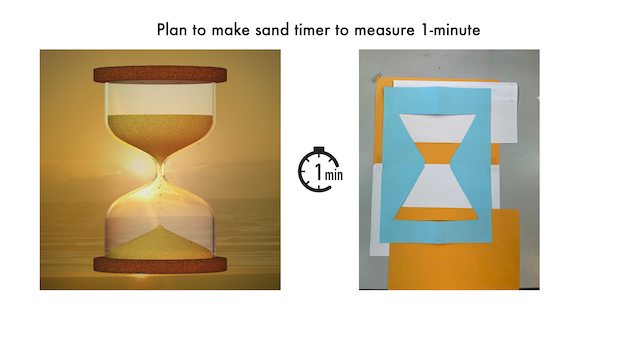• Prototype of sand timer by 1-axis linear

Prototype the use of two moving boards to see if an hourglass movement can be achieved.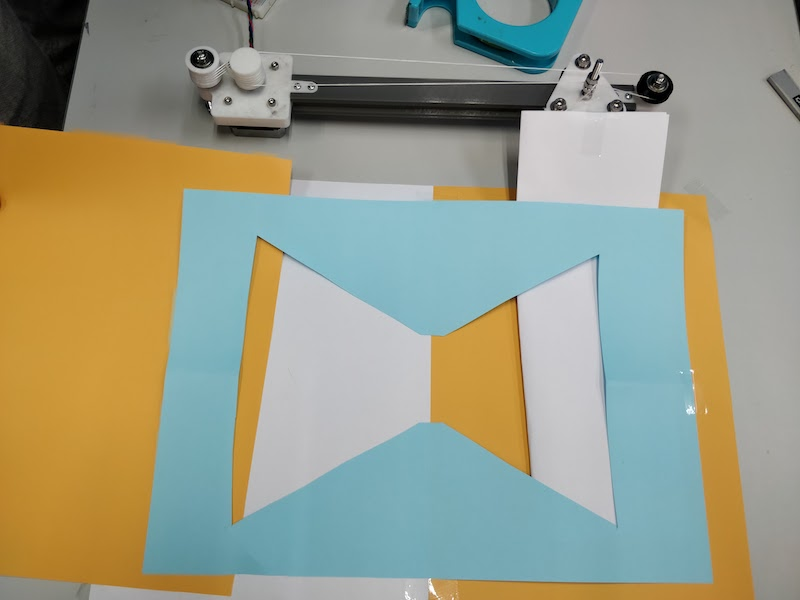• Finish to make the structure of vertical 1-axis linear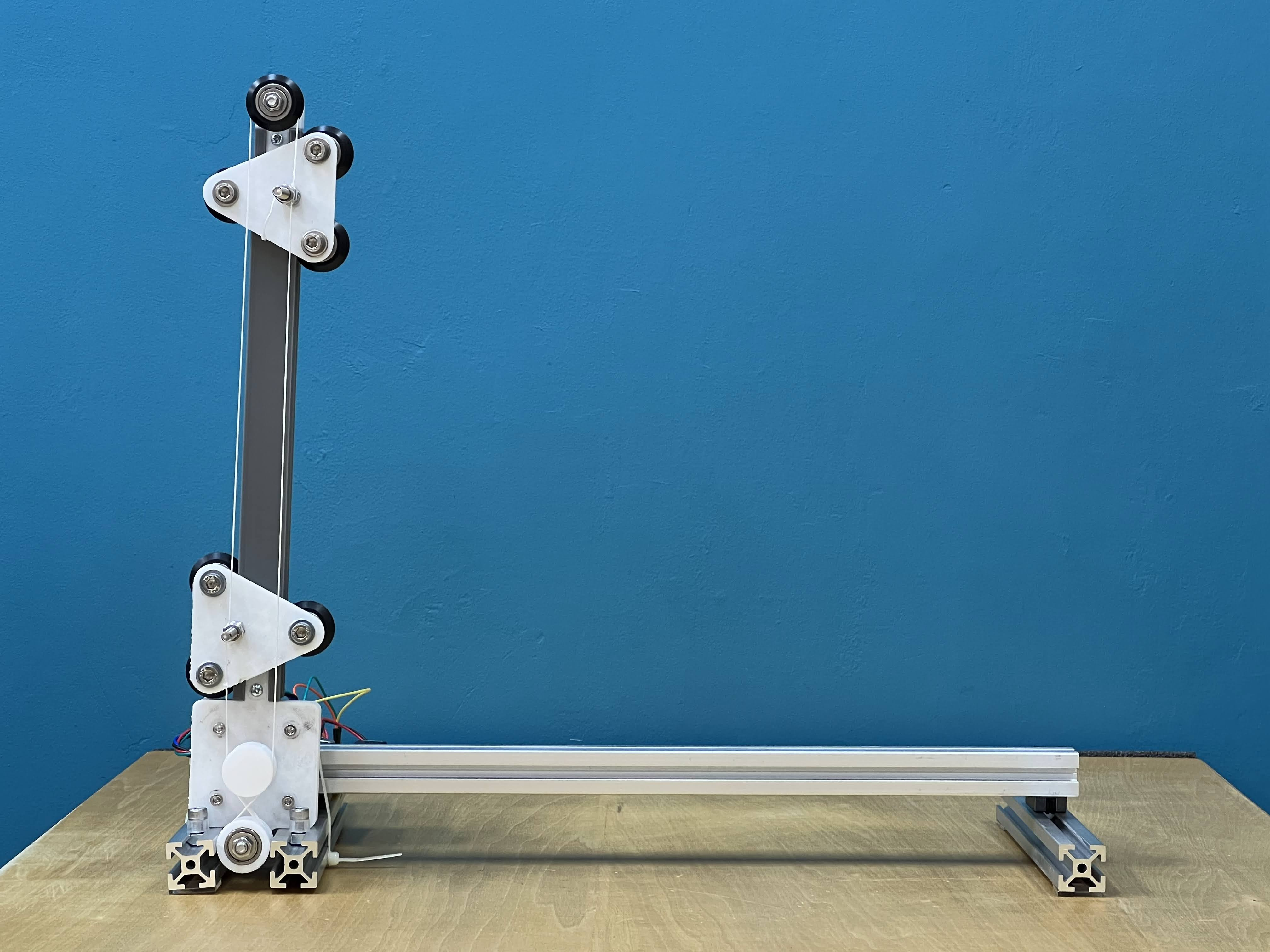• embed programming

1 minute count programme

``````/*
SilentStepStick TMC2208/TMC2209 Example
Rsense: 0.11 Ohm
Other examples/libraries can be found here:
https://github.com/teemuatlut/TMCStepper
https://github.com/trinamic/TMC-API
https://github.com/manoukianv/TMC2208Pilot
Example source code free to use.
*/

// Note: You also have to connect GND, 5V/VIO and VM.
//       A connection diagram can be found in the schematics.
#define EN_PIN    7 //enable (CFG6)
#define DIR_PIN   8 //direction
#define STEP_PIN  9 //step

void setup()
{
//set pin modes
pinMode(EN_PIN, OUTPUT);
digitalWrite(EN_PIN, HIGH); //deactivate driver (LOW active)

pinMode(DIR_PIN, OUTPUT);
digitalWrite(DIR_PIN, LOW); //LOW to CCW
//digitalWrite(DIR_PIN, HIGH); //HIGH to CW

pinMode(STEP_PIN, OUTPUT);
digitalWrite(STEP_PIN, LOW);

digitalWrite(EN_PIN, LOW); //activate driver
}

void loop()
{

delay(3000);

for (int i = 0; i <= 800; i++){  // center to xx cm in 30 sec

digitalWrite(DIR_PIN, HIGH); //LOW to CCW
//make steps
digitalWrite(STEP_PIN, HIGH);
delay(2);
digitalWrite(STEP_PIN, LOW);
delay(73);

}

delay(3000);

}
``````
• cut acrylic boards by laser cutter and set up them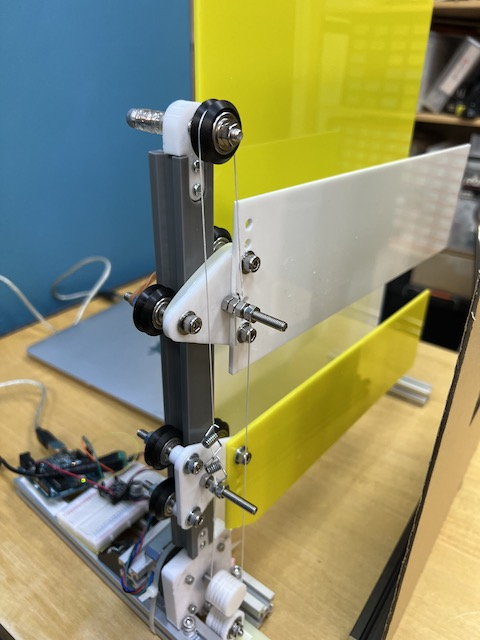mechanism of sand timer.• mechanism test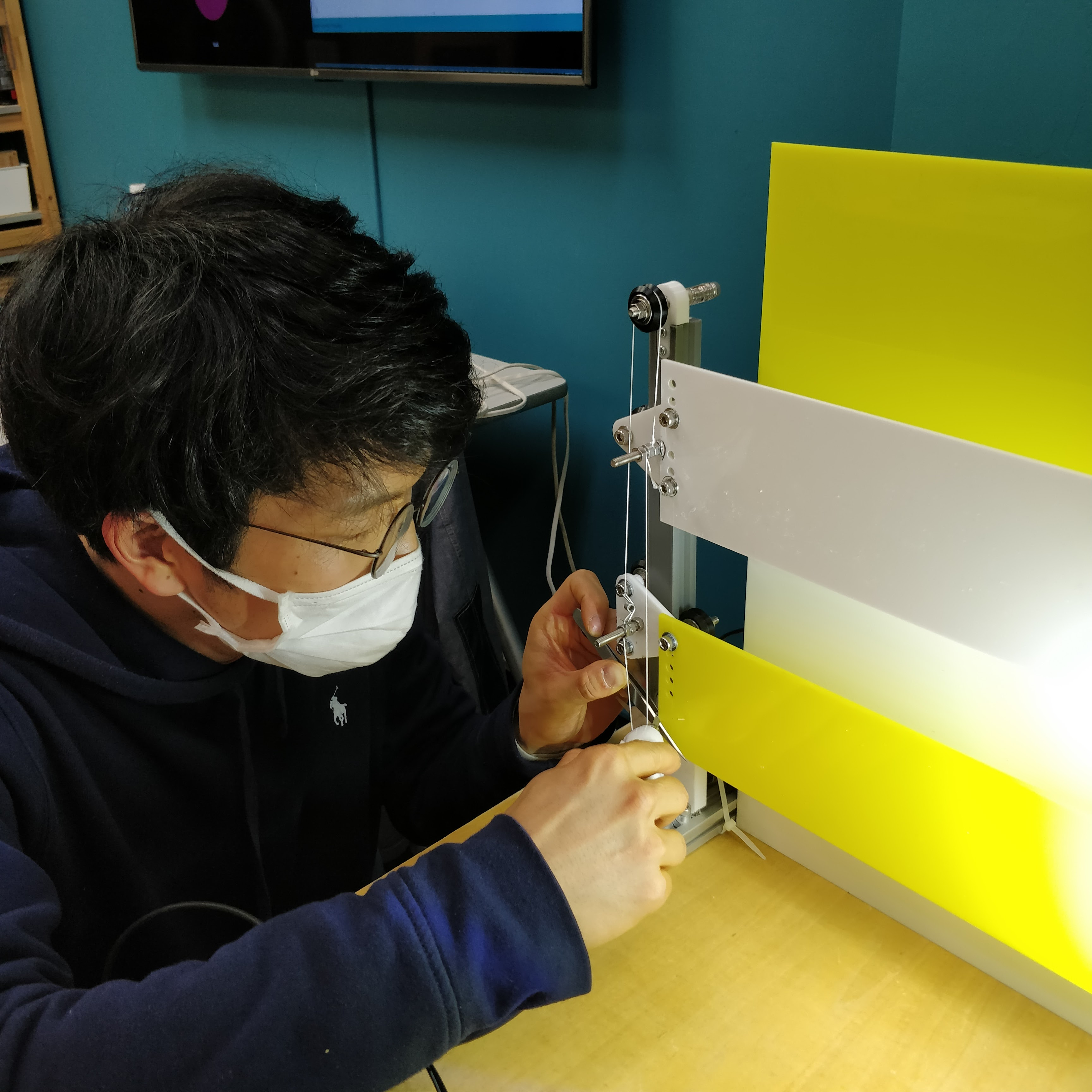• cut cardboard by laser cutter and put the acliryc board on the cardboard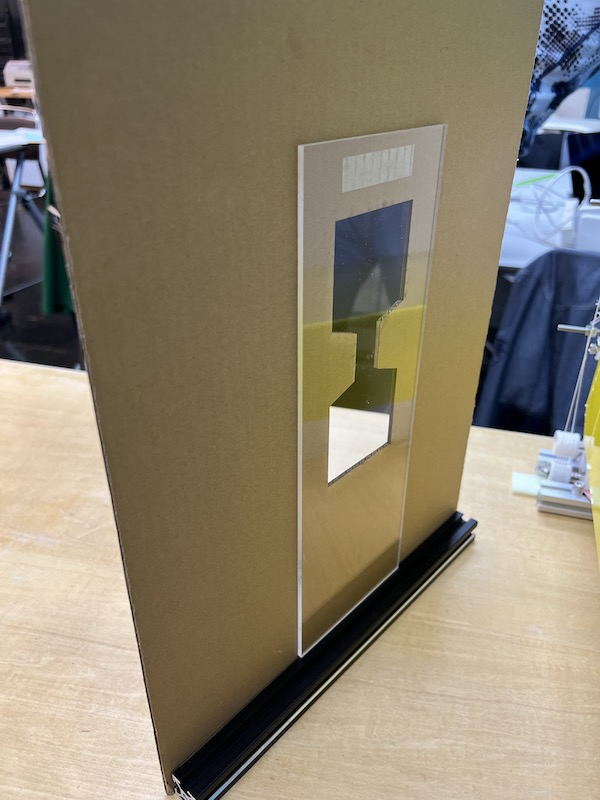• hero shot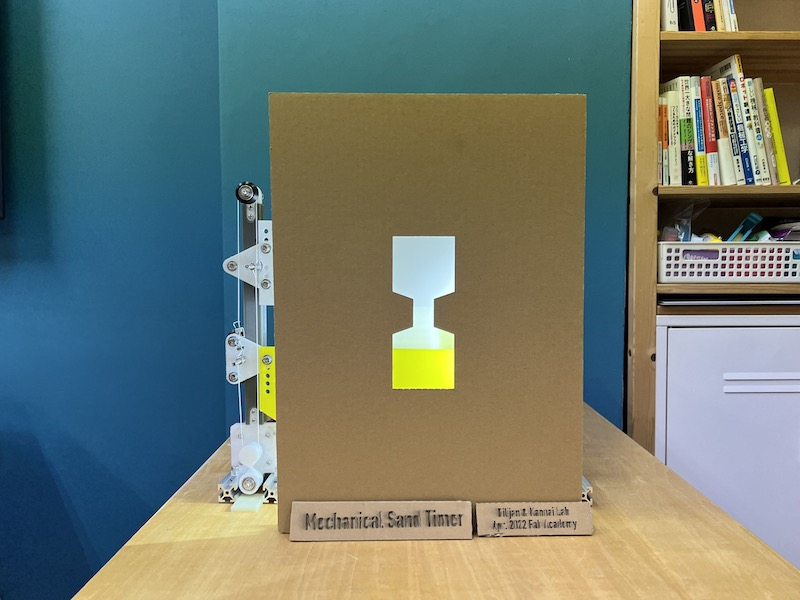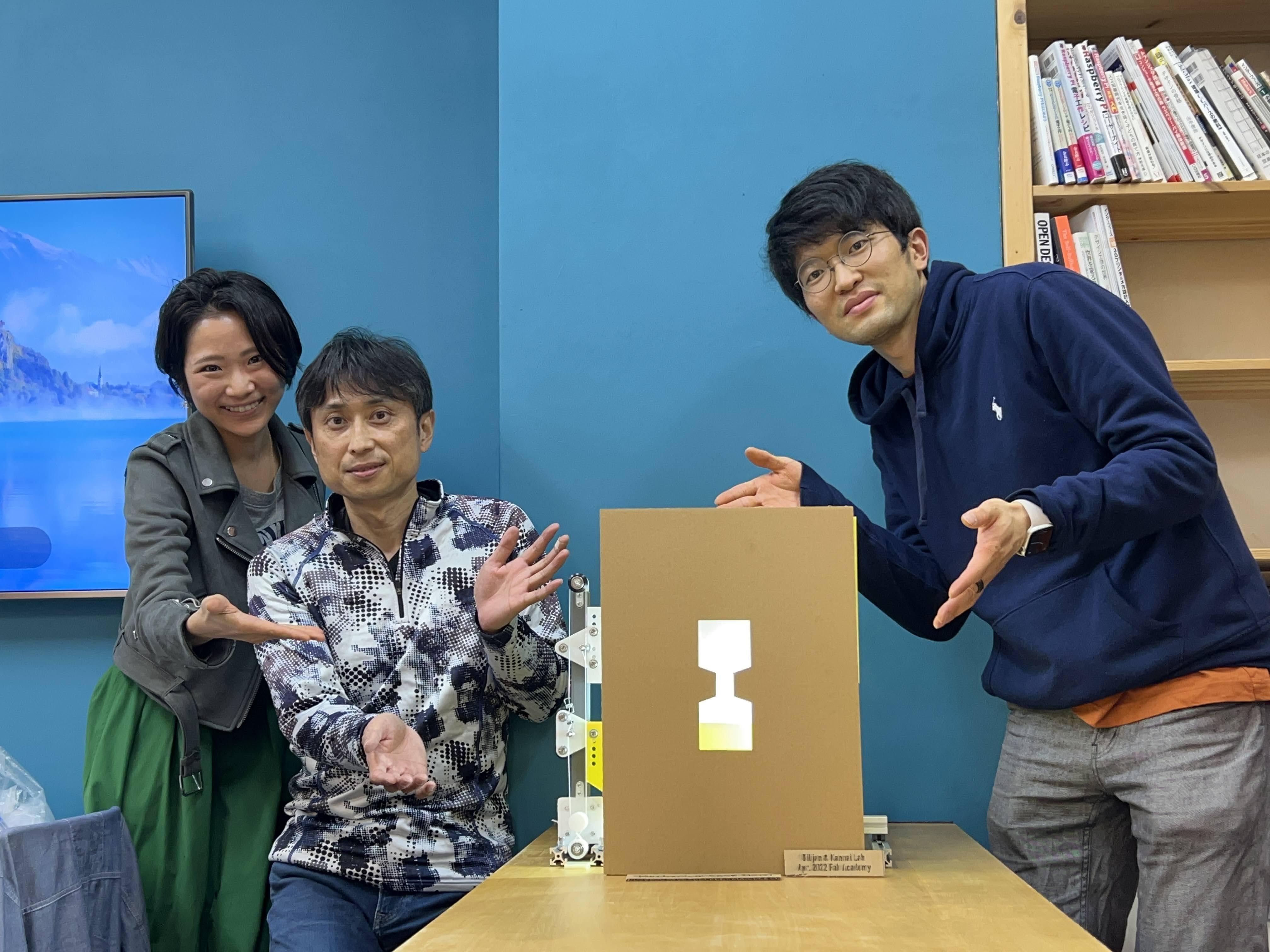Last update: June 28, 2022